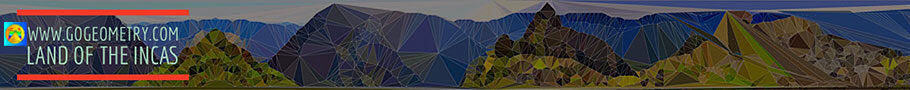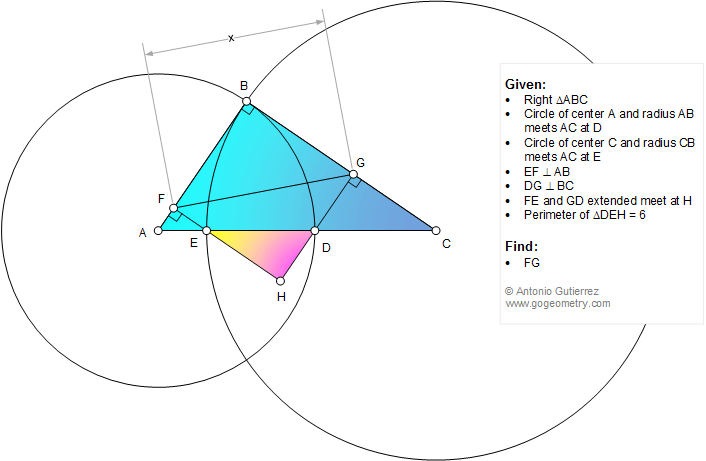# Online Math: Geometry Problem 1165: Right Triangle, Circle, Center, Radius, Cathetus, Perpendicular, 90 Degrees, Perimeter, Metric Relations. Level: School, College

< PREVIOUS PROBLEM  |  NEXT PROBLEM >

 The figure below shows a right triangle ABC (angle B = 90 degrees), the circle of center A and radius AB meets AC at D. The circle of center C and radius CB meets AC at E. EF and DG are perpendicular to AB and BC, respectively. FE and GD extended meet at H. If the perimeter of triangle DEH = 6, find the measure of FG.